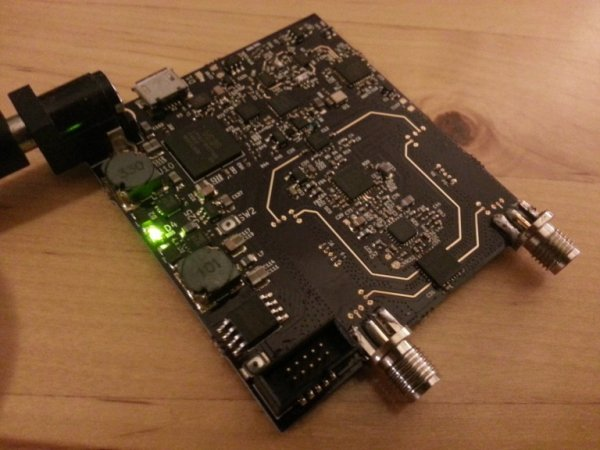# Arduino Wattmeter: Measure Voltage, Current and Power Consumption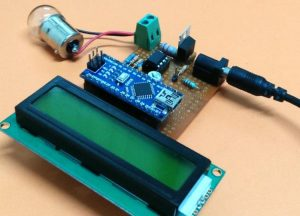As electronics engineers, we always depend upon meters/instruments to measure and analyse the working of a circuit. Starting with a simple multimeter to a complex power quality analysers or DSOs everything has their own unique applications. Most of these meters are readily available and can be purchased based on the parameters to be measured and their accuracy. But sometimes we might end up in a situation where we need to build our own meters. Say for instance you are working on a solar PV project and you would like to calculate the power consumption of your load, in such scenarios we can build our own Wattmeter using a simple microcontroller platform like Arduino.

Building your own meters not only bring down the cost of testing, but also gives us room to ease the process of testing. Like, a wattmeter built using Arduino can easily be tweaked to monitor the results on Serial monitor and plot a graph on Serial plotter or add an SD card to automatically log the values of voltage, current and power at pre-defined intervals. Sounds interesting right!? So let’s get started…

### Materials Required

• Arduino Nano
• LM358 Op-Amp
• 7805 Voltage regulator
• 16*2 LCD display
• 0.22 ohm 2Watt shunt resistor
• 10k Trimmer pot
• 10k,20k,2.2k,1k Resistors
• 0.1uF Capacitors
• Soldering kit (optional)

### Circuit Diagram

The complete circuit diagram of the arduino wattmeter project is given below.

For ease of understanding the arduino wattmeter circuit is split into two units. The upper part of the circuit is the measuring unit and the lower part of the circuit is the computation and display unit. For people who are new to this type of circuits followed the labels. Example +5V is label which means that all the pins to which label is connected to should be considered as they are connected together. Labels are normally used to make the circuit diagram look neat.

The circuit is designed to fit into systems operating between 0-24V with a current range of 0-1A keeping in mind the specification of a Solar PV. But you can easily extend the range once you understand the working of the circuit. The underlying principle behind the circuit is to measure the voltage across the load and current through it to calculate the power consumes by it. All the measured values will be displayed in a 16*2 Alphanumeric LCD.

Further below let’s split the circuit into small segments so that we can get a clear picture of how the circuit is indented to work.

Measuring Unit

The measuring unit consists of a potential divider to help us measure the voltage and a shut resistor with a Non-Inverting Op-amp is used to help us measure the current through the circuit. The potential divider part from the above circuit is shown below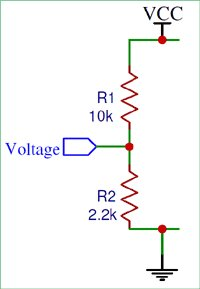Here the Input voltage is represent by Vcc, as told earlier we are designing the circuit for a voltage range from 0V to 24V. But a microcontroller like Arduino cannot measure such high values of voltage; it can only measure voltage from 0-5V. So we have to map (convert) the voltage range of 0-24V to 0-5V. This can be easily done by using a potential divider circuit as shown below. The resistor 10k and 2.2k together forms the potential divider circuit. The output voltage of a potential divider can be calculated using the below formulae. The same be used to decide the value of your resistors, you can use our online calculator to calculate value of resistor if you are re-designing the circuit.

Vout = (Vin × R2) / (R1 + R2)

The mapped 0-5V can be obtained from the middle part which is labelled as Voltage. This mapped voltage can then be fed to the Arduino Analog pin later.

Next we have to measure the current through the LOAD. As we know microcontrollers can read only analog voltage, so we need to somehow convert the value of current to voltage. It can be done by simply adding a resistor (shunt resistor) in the path which according to Ohm’s law will drop a value of voltage across it that is proportional to the current flowing through it. The value of this voltage drop will be very less so we use an op-amp to amplify it. The circuit for the same is shown below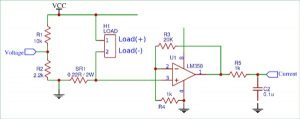Here the value of shunt resistor (SR1) is 0.22 Ohms. As said earlier we are designing the circuit for 0-1A so based on Ohms law we can calculate the voltage drop across this resistor which will be around 0.2V when a maximum of 1A current is passing through the load. This voltage is very small for a microcontroller to read, we use an Op-Amp in Non-Inverting Amplifier mode to increase the voltage from 0.2V to higher level for the Arduino to read.

The Op-Amp in Non-Inverting mode is shown above. The amplifier is designed to have a gain of 21, so that 0.2*21 = 4.2V. The formulae to calculate the gain of the Op-amp is given below, you can also use this online gain calculator to get the value of your resistor if you are re-designing the circuit.

Gain = Vout / Vin = 1 + (Rf / Rin)

Here in our case the value of Rf is 20k and the value of Rin is 1k which gives us a gian value of 21. The amplified voltage form the Op-amp is then given to a RC filter with resistor 1k and a capacitor 0.1uF to filter any noise that is coupled. Finally the voltage is then fed to the Arduino analog pin.

The last part that is left in the measuring unit is the voltage regulator part. Since we will give a variable input voltage we need a regulated +5V volt for the Arduino and the Op-amp to operate. This regulated voltage will be provided by the 7805 Voltage regulator. A capacitor is added at the output to filter the noise.### Computation and display unit

In the measuring unit we have designed the circuit to convert the Voltage and Current parameters into 0-5V which can be fed to the Arduino Analog pins. Now in this part of the circuit we will connect these voltage signals to Arduino and also interface a 16×2 alphanumeric display to the Arduino so that we can view the results. The circuit for the same is shown below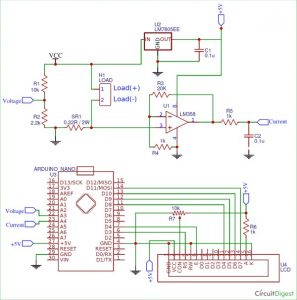As you can see the Voltage pin is connected to Analog pin A3 and the current pin is connected to Analog pin A4. The LCD is powered from the +5V from the 7805 and is connected to the digital pins of Arduino to work in 4-bit mode. We have also used a potentiometer (10k) connected to Con pin to vary the contrast of the LCD.

### Programming the Arduino

Now that we have a good understanding of the hardware, let us open the Arduino and start programming. The purpose of the code is to read the analog voltage on pin A3 and A4 and calculate the Voltage, Current and Power value and finally display it on the LCD screen. The complete program to do the same is given at the end of the page which can be used as such for the hardware discussed above. Further the code is split into small snippets and explained.

As all programs we begin with, defining the pins that we have used. In out project the A3 and A4 pin is used to measure voltage and current respectively and the digital pins 3,4,8,9,10 and 11 is used for interfacing the LCD with Arduino

const int rs = 3, en = 4, d4 = 8, d5 = 9, d6 = 10, d7 = 11; //Mention the pin number for LCD connection
LiquidCrystal lcd(rs, en, d4, d5, d6, d7);

We also have included a header file called liquid crystal to interface the LCD with Arduino. Then inside the setup function we initialise the LCD display and display an intro text as “Arduino Wattmeter” and wait for two seconds before clearing it. The code for the same is shown below.

void setup() {
lcd.begin(16, 2); //Initialise 16*2 LCD
lcd.print(” Arduino Wattmeter”); //Intro Message line 1
lcd.setCursor(0, 1);
lcd.print(“-Circuitdigest”); //Intro Message line 2
delay(2000);
lcd.clear();
}

Inside the main loop function, we use the analog read function to read the voltage value from the pin A3 and A4. As we know the Arduino ADC output value from 0-1203 since it has a 10-bit ADC. This value has to be then converted to 0-5V which can be done by multiplying with (5/1023). Then again earlier in the hardware we have mapped the actual value of voltage from 0-24V to 0-5V and the actual value of current form 0-1A to 0-5V. So now we have to use a multiplier to revert these values back to actual value. This can be done by multiplying it with a multiplier value. The value of the multiplier can either be calculated theoretically using the formulae provided in hardware section or if you have a known set of voltage and current values you can calculate it practically. I have followed the latter option because it tends to be more accurate in real time. So here the value of multipliers is 6.46 and 0.239. Hence the code looks like below

Voltage_Value = Voltage_Value * (5.0/1023.0) * 6.46;
Current_Value = Current_Value * (5.0/1023.0) * 0.239;

### How to measure with more accuracy?

The above way of calculating the value of Actual Voltage and current will work just fine. But suffers from one drawback, that is the relationship between the measured ADC voltage and actual voltage will not be linear hence a single multiplier will not give very accurate results, the same applied for current as well.

So to improve the accuracy we can plot of set of measured ADC values with actual vales using a known set of values and then use that data to plot a graph and derive the multiplier equation using the linear regression method. You can refer the Arduino dB meter in which I have used a similar method.

Finally, once we have calculated the value of actual voltage and actual current through the load, we can calculate the Power using the formulae (P=V*I). Then we display all the three values on the LCD display using the code below.

lcd.setCursor(0, 0);
lcd.print(“V=”); lcd.print(Voltage_Value);
lcd.print(” “);
lcd.print(“I=”);lcd.print(Current_Value);

float Power_Value = Voltage_Value * Current_Value;

lcd.setCursor(0, 1);
lcd.print(“Power=”); lcd.print(Power_Value);

### Working and Testing

For the sake of tutorial I have used a perf board to solder all the components as shown in the circuit. I have used a Phoenix screw terminal to connect the load and normal DC barrel Jack to connect my power source. The Arduino Nano board and the LCD are mounted on a Female Bergstik so that they can be re-used if required later.

After getting the hardware ready, upload the Arduino code to your Nano board. Adjust the trimmer pot to control the contrast level of the LCD until you see a clear intro text. To test the board connect the load to the screw terminal connector and the source to the Barrel jack. The source voltage should be more than 6V for this project to work, since the Arduino required +5V to operate. IF everything is working fine you should see the value of Voltage across the load and the current through it displayed in the first line of the LCD and the calculated power displayed on the second line of the LCD as shown below.The fun part of building something lies in testing it to check how far it will work properly. To do that I have used 12V automobile indicator bubs as load and the RPS as source. Since the RPS itself can measure and display the value of current and voltage it will be easy for us to cross check the accuracy and performance of our circuit. And yes, I also used my RPS to calibrate my multiplier value so that I get close to accurate value.

The complete working can be found at the video given at the end of this page. Hope you understood the circuit and program and learnt something useful. If you have any problem in getting this to work post it on the comment section below or write on our forums for more technical help.

This Arduino based Wattmeter project has many more upgrades that can be added to increase the performance to auto data logging, plotting graph, notifying over voltage or over current situations etc. So stay curious and let me know what you would use this for.

Code

/*
* Wattmeter for Solar PV using Arduino
* Dated: 27-7-2018
* Website: www.circuitdigest.com
*
* Power LCD and circuitry from the +5V pin of Arduino whcih is powered via 7805
* LCD RS -> pin 2
* LCD EN -> pin 3
* LCD D4 -> pin 8
* LCD D5 -> pin 9
* LCD D6 -> pin 10
* LCD D7 -> pin 11
* Potetnital divider to measure voltage -> A3
* Op-Amp output to measure current -> A4
*/

#include <LiquidCrystal.h>  //Default Arduino LCD Librarey is included

const int rs = 3, en = 4, d4 = 8, d5 = 9, d6 = 10, d7 = 11; //Mention the pin number for LCD connection
LiquidCrystal lcd(rs, en, d4, d5, d6, d7);

void setup() {
lcd.begin(16, 2); //Initialise 16*2 LCD

lcd.print(” Arduino Wattmeter”); //Intro Message line 1
lcd.setCursor(0, 1);
lcd.print(”  With Arduino  “); //Intro Message line 2

delay(2000);
lcd.clear();

}

void loop() {

Voltage_Value = Voltage_Value * (5.0/1023.0) * 6.46;
Current_Value = Current_Value * (5.0/1023.0) * 0.239;

lcd.setCursor(0, 0);
lcd.print(“V=”); lcd.print(Voltage_Value);
lcd.print(”  “);
lcd.print(“I=”);lcd.print(Current_Value);

float Power_Value = Voltage_Value * Current_Value;

lcd.setCursor(0, 1);
lcd.print(“Power=”); lcd.print(Power_Value);

delay(200);
}

#### This Post / Project can also be found using search terms:

• wattmètre arduino project
• arduino 24v wattmeter
• arduino code for measuring voltage and current
• arduino lcd wattmeter